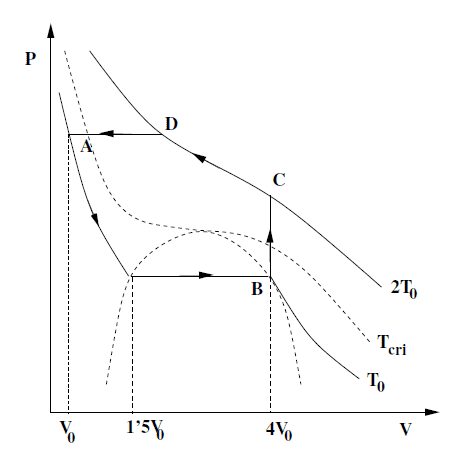# Isobaric process for a Van der Waals gas

Luis Obis

## Homework Statement

[/B]
You are asked to calculate changes in internal energy, entropy, heat transferred and work done for each of the following process. Also you are asked to calculate "the latent heat for the isotherm in the figure".

We know the a and b parameters which characterize the VdW gas since you can get them from the shape of the isotherms assuming you know the volume V0 and the critical temperature Tcri.

Assume ##N = 1 mol##.## Homework Equations

Pressure for the VdW gas (N = 1):

##P = \frac{RT}{V-b}-\frac{a}{V^2}##

## The Attempt at a Solution

I have done as asked for every process but for D->A.

The problem is that we don't know the volume at D. We could calculate it using the VdW equation and using the fact that the pressures at A and D are equal, however this will give us a third degree equation which we could solve analitcally but I don't think this would be the best answer. Any ideas how to proceed?

For the latent heat I have done as follows:

##L = T \Delta S##

Where in this case ##T## would be ##T_0## and ##\Delta S## would be the change in entropy from 1.5V0 to 4V0 in the isotherm ##T = T_0##. Is this correct?

Thanks you very much for any help!